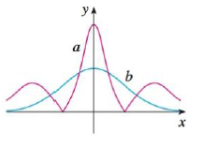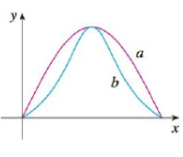Chapter 13.3, Problem 38E

Chapter
Section
Textbook Problem

# Two graphs, a and b, are shown. One is a curve y = f(x) and the other is the graph of its curvature function y = k(x). Identify each curve and explain your choices.38.39.To determine

To identify: Each curve in the given graph.

Explanation

The curve a has a maximum value, whereas curve b is turning more sharply for a value of x. Similarly, the curve a is almost zero, whereas curve b is approximately straight for a specific value of x. Hence, curve a must be a graph of the curvature of function y=k(x) and curve b is a curve y=f(x)

### Still sussing out bartleby?

Check out a sample textbook solution.

See a sample solution

#### The Solution to Your Study Problems

Bartleby provides explanations to thousands of textbook problems written by our experts, many with advanced degrees!

Get Started

#### In problems 63-73, factor each expression completely. 66.

Mathematical Applications for the Management, Life, and Social Sciences

#### In Exercises 116, determine whether the argument is valid. pqqrpr

Finite Mathematics for the Managerial, Life, and Social Sciences

#### ∫x−4dx = −4x−5 + C

Study Guide for Stewart's Single Variable Calculus: Early Transcendentals, 8th